# Complex Numbers

 The unit of imaginary numbers isand is generally designated by the letter i (or j). Many laws which are true for real numbers are true for imaginary numbers as well. Thus. Matlab recognizes the letters i and j as the imaginary number.

A complex number 3 + 10i may be input as 3 + 10i or 3 + 10*i in Matlab (make sure not to use i as a variable).

In the complex number a + bi, a is called the real part (in Matlab, real(3+5i) = 3) and b is the coefficient of the imaginary part (in Matlab, imag(4-9i) = -9). When a = 0, the number is called a pure imaginary. If b = 0, the number is only the real number a. Thus, complex numbers include all real numbers and all pure imaginary numbers.

The conjugate of a complex a + bi is a - bi. In Matlab, conj(2 - 8i) = 2 + 8i.

To add (or subtract) two numbers, add (or subtract) the real parts and the imaginary parts separately. For example:
(a+bi) + (c-di) = (a+c)+(b-d)i

In Matlab, it's very easy to do it:
>> a = 3-5i
a =
3.0000 - 5.0000i

>> b = -9+3i
b =
-9.0000 + 3.0000i

>> a + b
ans =
-6.0000 - 2.0000i

>> a - b
ans =
12.0000 - 8.0000i

To multiply two numbers, treat them as ordinary binomials and replace i2 by -1. To divide two complex nrs., multiply the numerator and denominator of the fraction by the conjugate of the denominator, replacing again i2 by -1.

Don't worry, in Matlab it's still very easy (assuming same a and b as above):
>> a*b
ans =
-12.0000 +54.0000i

>> a/b
ans =
-0.4667 + 0.4000i

 Employing rectangular coordinate axes, the complex nr. a+bi is represented by the point whose coordinates are (a,b). We can plot complex nrs. this easy way. To plot numbers (3+2i), (-2+5i) and (-1-1i), we can write the following code in Matlab.

Example - Plotting Complex Numbers:

% Enter each coordinate (x,y) separated by commas
% Each point is marked by a blue circle ('bo')
plot(3,2,'bo', -2,5,'bo', -1,-1,'bo')

% You can define the limits of the plot [xmin xmax ymin ymax]
axis([-3 4 -2 6])

% Add some labels to explain the plot
xlabel('x (real axis)')
ylabel('y (imaginary axis)')

% Activate the grid
grid on

And you get: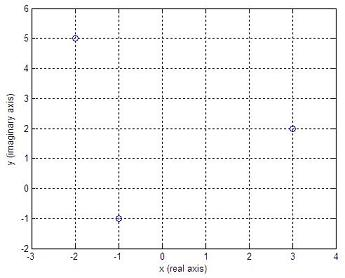# Polar Form of Complex Nrs.

In the figure below,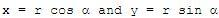.
Then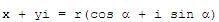.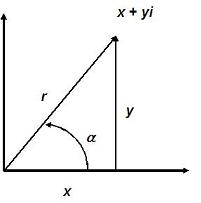Then x + yi is the rectangular form and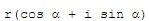is the polar form of the same complex nr. The distance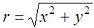is always positive and is called the absolute value or modulus of the complex number. The angle is called the argument or amplitude of the complex number.

In Matlab, we can effortlessly know the modulus and angle (in radians) of any number, by using the 'abs' and 'angle' instructions. For example:

a = 3-4i
magnitude = abs(a)
ang = angle(a)

a =
3.0000 - 4.0000i

magnitude =
5

ang =
-0.9273

# De Moivre's Theorem

The nth power ofis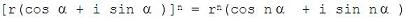This relation is known as the De Moivre's theorem and is true for any real value of the exponent. If the exponent is 1/n, then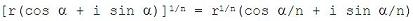.

It's a good idea if you make up some exercises to test the validity of the theorem.

# Roots of Complex Numbers in Polar Form

If k is any integer,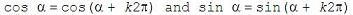.

Then,Any number (real or complex) has n distinct nth roots, except zero. To obtain the n nth roots of the complex number x + yi, or, let k take on the successive values 0, 1, 2, ..., n-1 in the above formula.

Again, it's a good idea if you create some exercises in Matlab to test the validity of this affirmation. Working with complex or imaginary numbers is easy with Matlab.

From 'Complex Numbers' to home

From 'Complex Numbers' to 'Matlab Examples'

 Coordinaate Conversion